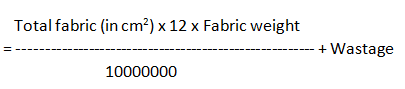# Consumption of T-Shirt and Polo Shirt by Apparel Merchandiser

We know that fabric consumption is the most important task for apparel merchandiser. Maximum time profit or loss depends on the total consumption of fabric in an order. So accurate consumption will reduce fabric wastage which will be beneficial to make cost effective apparels. So apparel merchandiser should have proper knowledge about consumption. T-shirt and polo shirt are very common apparels. These two type apparels are ordered in bulk amount by foreign garment buyers. In this article I will discuss how apparel merchandisers do consumption of t-shirt and polo shirt. I think this article will be very helpful for trainee merchandisers.

### Consumption Calculation of a T-Shirt:

A measurement chart of a t-shirt is given below table:

 Points of measurement Unit (cm) Body length 60 ½ Chest 45 Sleeve length 20 Armhole circumference 40 Neck height 2 Neck circumference 36 Body fabric weight (gsm) 140 Rib fabric weight (gsm) 175

Front and back:

Length x Width x 2

= (Body length + Sewing Allowance, SA) x (1/2 Chest + SA) x 2

= (60+3) x (45+2) x 2

= 5922 cm2

Sleeve:

Length x Width x 2

= (Sleeve length + SA) x (Armhole circumference + SA) x 2

= (20+3) x (40+2) x 2

= 1932 cm2

Total Fabric (in cm2)

= Front and back + Sleeve

= 5922 + 1932

=7854 cm2

Fabric consumption per Dozen,784 x 12 x 140
= —————————– + 10%
10000000

= 1.45 kg/dzn

Neck binding (Rib fabric),

Length x 2 x Width

= (Neck height + SA) x 2 x (Neck circumference + SA)

= (2+1) x 2 x (36+2)

= 228 cm2

Fabric consumption per Dozen,228 x 12 x 175
= ———————————————– + 10%
10000000

= 0.06 kg/dzn

Body fabric = 1.45 kg/dzn

Rib fabric = 0.06 kg/dzn

Polo Shirt Consumption:

A measurement chart of a polo shirt is given below:

 Points of measurement Unit (cm) Body length 62 ½ Chest 48 Sleeve length 22 Armhole circumference 40 Collar height 5 Collar width 24 Cuff height 2.5 Body fabric weight (gsm) 180 Rib fabric weight (gsm) 230

Front and Back:

Length x Width x 2

= (Body length + SA) x (½ Chest + SA) x 2

= (62 + 3) x (48+2) x 2

= 6500 cm2

Sleeve:

Length x Width x 2

= (Sleeve length – Sleeve Rib height + SA) x (Armhole circumference + SA) x 2

= (22 – 2.5 + 2) x (40+2) x 2

= 1806 cm2

Total Fabric (in cm2) = Front and Back + Sleeve

= 6500 + 1806

= 8306 cm2

Fabric consumption per Dozen,8306 x 12 x 180
= —————————————- + 10%
10000000

= 1.97 kg/dzn

In addition, polo shirt requires two flat knitted cuffs and one flat knitted collar.

Collar:

Length x Width

= (Collar height + SA) x Collar width (no allowance, as it is open)

= (5+1) x 24

= 144 cm2

Cuff:

Length x Width x 2

= (Cuff height + SA) x (Sleeve opening + SA) x 2

= (2.5+1) x (17.5+2) x 2

= 136.5 cm2

Total Rib fabric (in cm2) = Collar + Cuff

= 144 + 136.5

= 280.5 cm2

Rib Fabric consumption per Dozen,…..280.5 x 12 x 230
= ——————————— + 10%
………10000000

= 0.09 kg/dzn